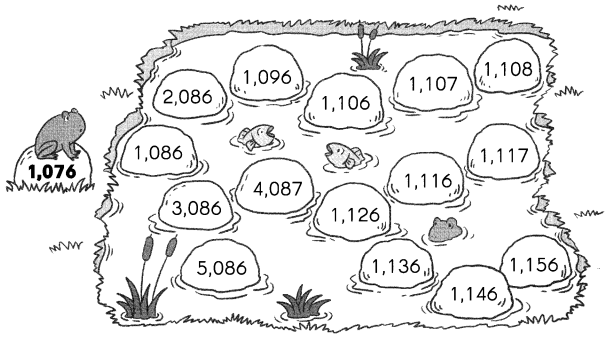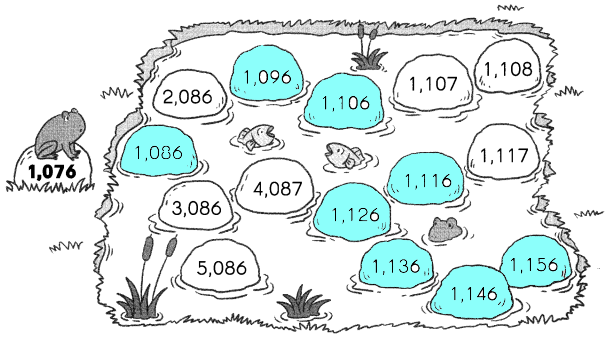# Math in Focus Grade 3 Chapter 1 Practice 1 Answer Key Counting

Go through the Math in Focus Grade 3 Workbook Answer Key Chapter 1 Practice 1 Counting to finish your assignments.

## Math in Focus Grade 3 Chapter 1 Practice 1 Answer Key Counting

Write each number shown.

Example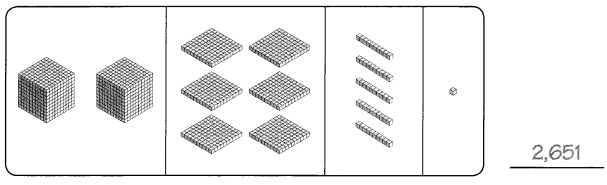Question 1.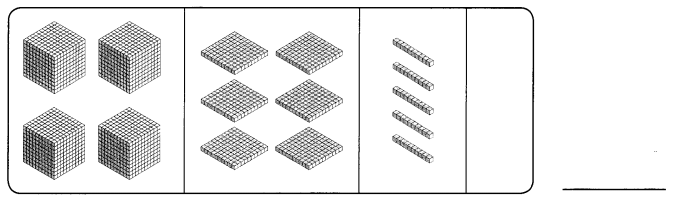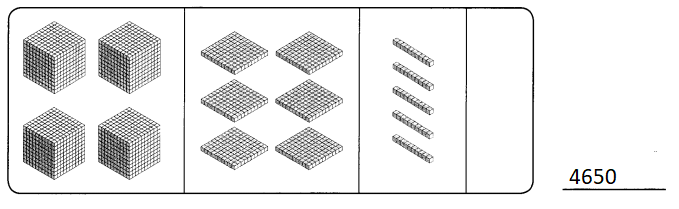Explanation:
Each cube shows thousand and each square shows hundred
each line shows tens and dot shows the ones

Question 2.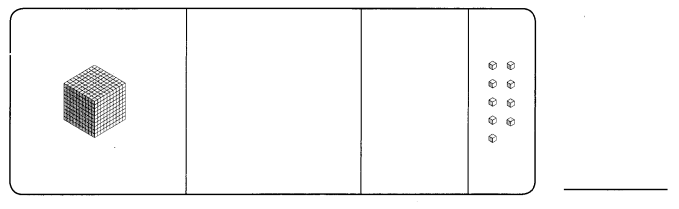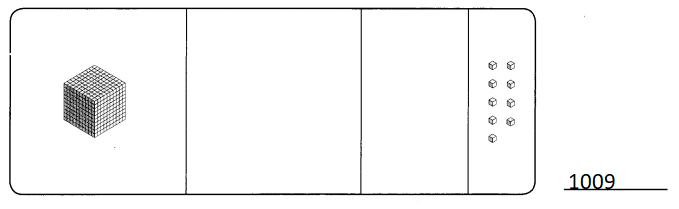Explanation:
Each cube shows thousand and each square shows hundred
each line shows tens and dot shows the ones

Write each number in word form.

Question 3.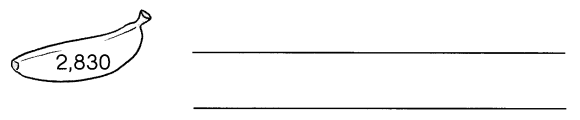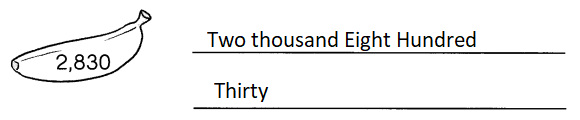Explanation:
The given number written in the expanded form

Question 4.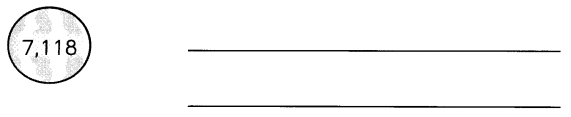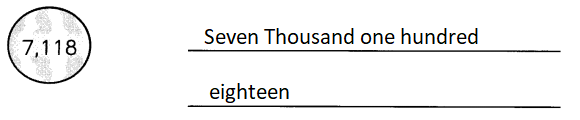Explanation:
The given number written in the expanded form

Question 5.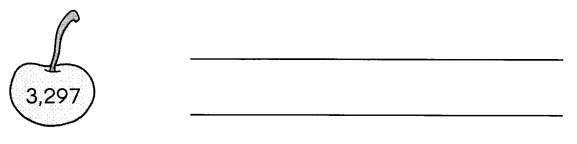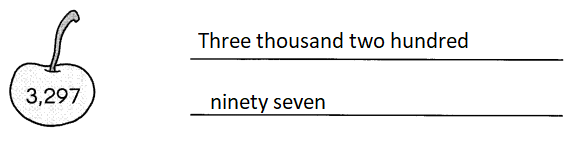Explanation:
The given number written in the expanded form

Question 6.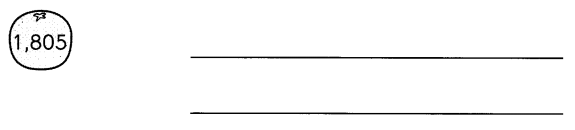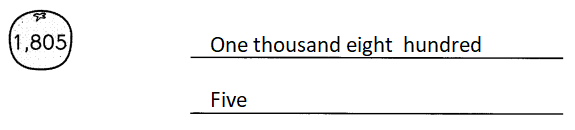Explanation:
The given number written in the expanded form

Question 7.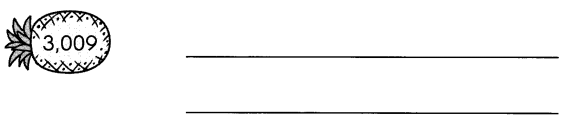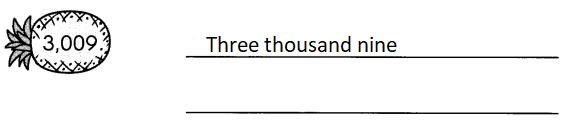Explanation:
The given number written in the expanded form

Question 8.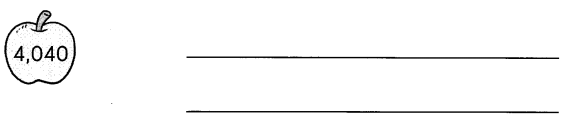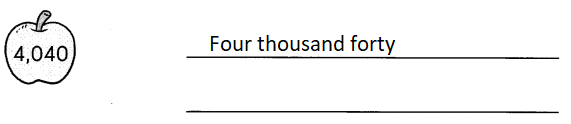Explanation:
The given number written in the expanded form

Write each number in the box.

Question 9.
nine thousand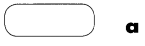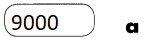Explanation:
The given expanded form converted in to the standard form

Question 10.
one thousand, four hundred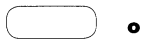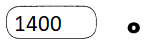Explanation:
The given expanded form converted in to the standard form

Question 11.
six thousand, eight hundred thirty-five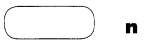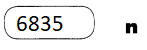Explanation:
The given expanded form converted in to the standard form

Question 12.
three thousand, two hundred sixty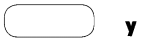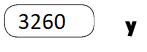Explanation:
The given expanded form converted in to the standard form

Question 13.
three thousand, two hundred six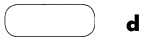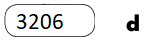Explanation:
The given expanded form converted in to the standard form

Question 14.
five thousand, sixty-eight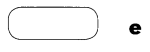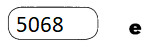Explanation:
The given expanded form converted in to the standard form

Question 15.
two thousand, seventy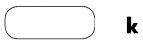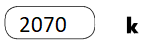Explanation:
The given expanded form converted in to the standard form

Question 16.
five thousand, nine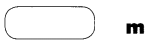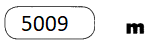Explanation:
The given expanded form converted in to the standard form

Match the numbers to the letters.

Question 17.
Who ate the banana?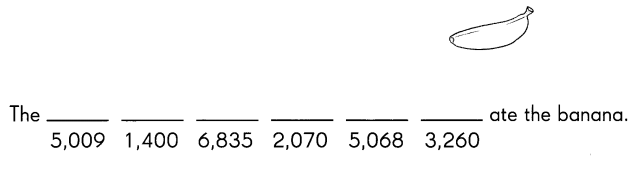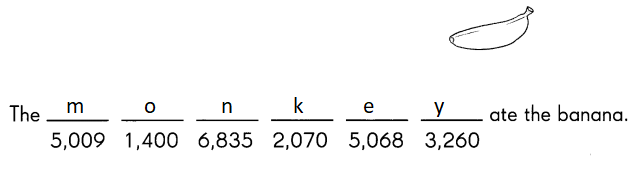Explanation:
According to the above answers the monkey ate the banana

Count on by ones, tens, hundreds, or thousands.
Fill in the missing numbers.

Question 18.
2,065 2,066 ___ 2,068 _____ 2,070
2,065 2,066 2,067 2,068 2,069 2,070
Explanation:
In the above pattern the number increase in once place by 1

Question 19.
____ _____ 7,543 7,553 7,563 ____
7,523 7,533 7,543 7,553 7,563 7,573
Explanation:
In the above pattern the number increase in tens place by 10

Question 20.
3,307 3,407 3,507 ___ ____ ____
3,307 3,407 3,507 3,607 3,707 3,807
Explanation:
In the above pattern the number increase in hundreds place by 100

Question 21.
3,654 4,654 ___ ____ 7,654 8,654
3,654 4,654 5,654 6,654 7,654 8,654
Explanation:
In the above pattern the number increase in thousands place by 1000

Count by tens. Color the rocks the frog jumps on.

Question 22.